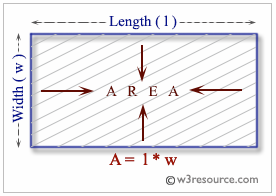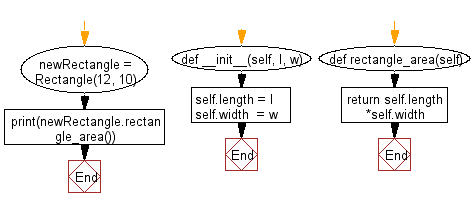﻿ Python: A class constructed by a length and width and a method which will compute the area of a rectangle - w3resource# Python: A class constructed by a length and width and a method which will compute the area of a rectangle

## Python Class: Exercise-10 with Solution

Write a Python class named Rectangle constructed by a length and width and a method which will compute the area of a rectangle.

Python: Area of a rectangle

In Euclidean plane geometry, a rectangle is a quadrilateral with four right angles. To find the area of a rectangle, multiply the length by the width.
A rectangle with four sides of equal length is a square.
Following image represents the area of a rectangle.Sample Solution:

Python Code:

``````class Rectangle():
def __init__(self, l, w):
self.length = l
self.width  = w

def rectangle_area(self):
return self.length*self.width

newRectangle = Rectangle(12, 10)
print(newRectangle.rectangle_area())
```
```

Sample Output:

```120
```

Flowchart:## Visualize Python code execution:

The following tool visualize what the computer is doing step-by-step as it executes the said program:

Python Code Editor:

What is the difficulty level of this exercise?

Test your Programming skills with w3resource's quiz.

﻿

## Python: Tips of the Day

The Zip() Function:

```>>> students = ('John', 'Mary', 'Mike')
>>> ages = (15, 17, 16)
>>> scores = (90, 88, 82, 17, 14)
>>> for student, age, score in zip(students, ages, scores):
...     print(f'{student}, age: {age}, score: {score}')
...
John, age: 15, score: 90
Mary, age: 17, score: 88
Mike, age: 16, score: 82
>>> zipped = zip(students, ages, scores)
>>> a, b, c = zip(*zipped)
>>> print(b)
(15, 17, 16)
```